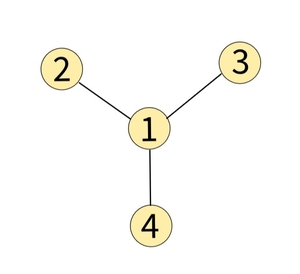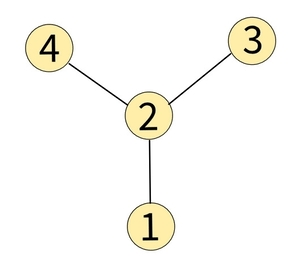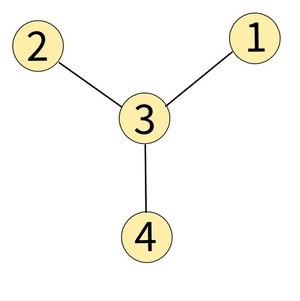Problem title
Difficulty
Avg time to solve

Find the Winner
Easy
10 mins
Custom Sort String
Easy
10 mins
Battalions and tanks
Moderate
10 mins
Mirror String
Easy
15 mins
Find the K-th Smallest Element in Array
Easy
10 mins
Median of a row wise sorted matrix
Moderate
30 mins
Minimum Number Of Swaps For Bracket Balancing
Moderate
15 mins
Minimum Rotations
Easy
10 mins
Rotate DLL
Moderate
10 mins
Minimum shift Operations
Moderate
30 mins1

# Find Center of Star Graph

Difficulty: EASY

Problem Statement

#### You are given a matrix ‘EDGES’ storing the information about the edges, where each row, ‘EDGES[i]’ contains two integers ‘U’ and ‘V’, which implies that there is an undirected edge between ‘U’ and ‘V’.

##### For Example :
``````For the given graph:
````````````Node 1 is connected to all other nodes. Hence node 1 is the centre of this star graph.
``````
##### Input Format :
``````The first line contains an integer 'T' which denotes the number of test cases.

The first line of each test case contains ‘M’, no of edges.

The following ‘M’ lines contain two integers ‘U’ and ‘V’ denoting there is an undirected edge between node ‘U’ and node ‘V’.
``````
##### Output Format :
``````For each test case, return the centre of the given star graph.

The first and only line of output of each test case prints the centre of a given star graph.
``````
##### Note :
``````You don't need to print anything, it has already been taken care of. Just implement the given function.
``````
##### Constraints :
``````1 ≤ T ≤ 10
3 ≤ M ≤ 10^4
M is always equal to N - 1
EDGES.length = M
1 ≤ U, V ≤ N

Time limit: 1 sec
``````
##### Sample Input 1 :
``````2
3
2 1
3 1
1 4
3
1 2
2 3
4 2
``````
##### Sample Output 1 :
``````1
2
``````
##### Explanation of Sample Output 1 :
``````In test case 1, the graph is shown below.
````````````Node 1 is connected to all other nodes. Hence node 1 is the centre of this star graph.

In test case 2, the graph is shown below.
````````````Node 2 is connected to all other nodes. Hence node 2 is the centre of this star graph.
``````
##### Sample Input 2 :
``````2
3
2 3
3 1
3 4
4
1 2
2 3
4 2
2 5
``````
##### Sample Output 2 :
``````3
2
``````
##### Explanation of Sample Output 2 :
``````In test case 1, the graph is shown below.
````````````Node 3 is connected to all other nodes. Hence node 3 is the centre of this star graph.

In test case 2, the graph is shown below.
````````````Node 2 is connected to all other nodes. Hence node 2 is the centre of this star graph.
``````Console